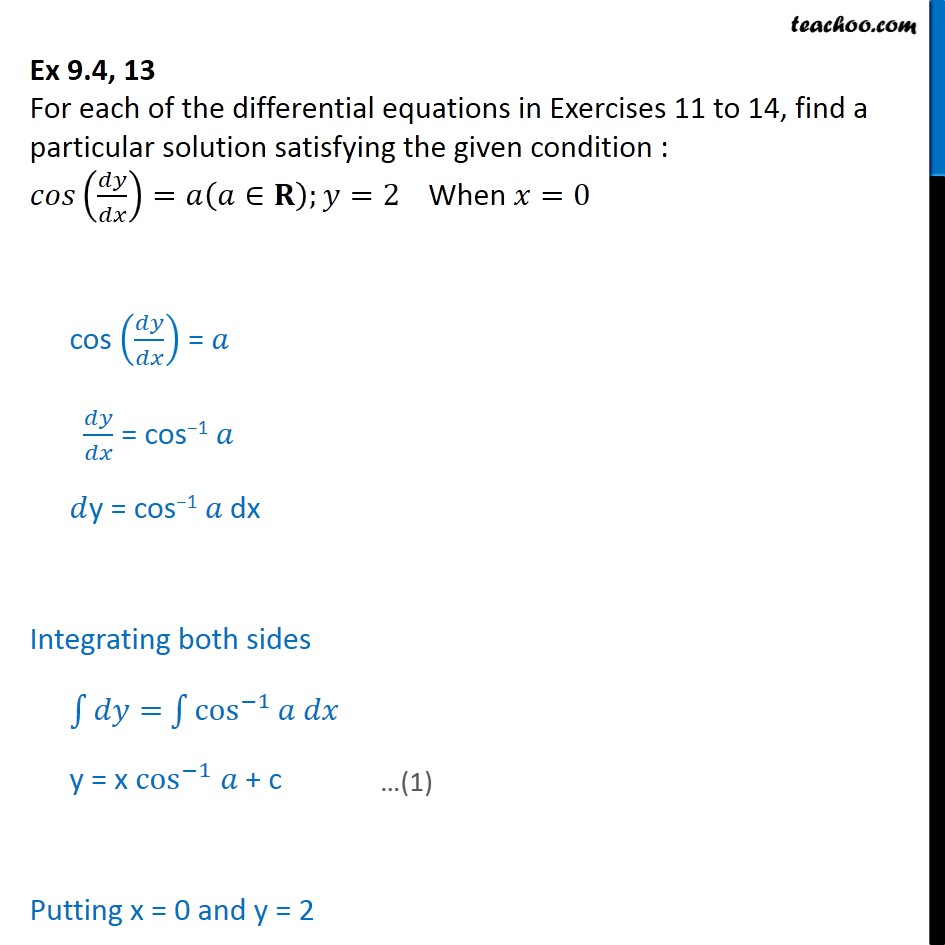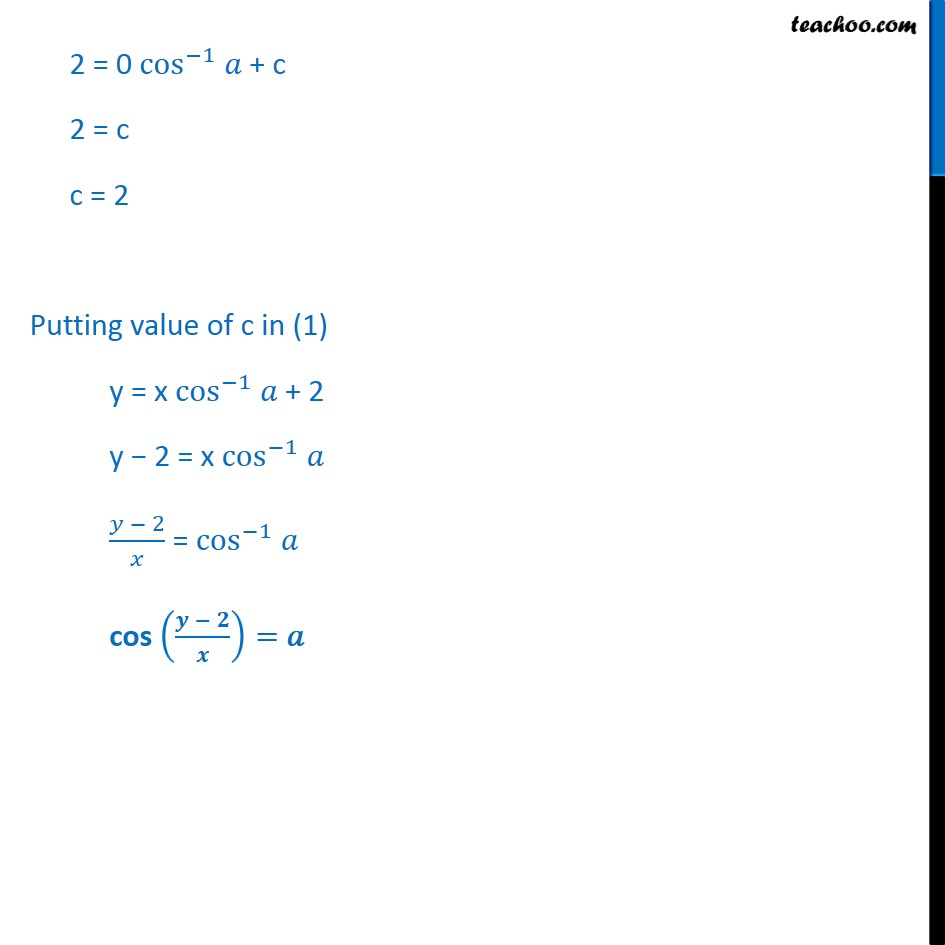Ex 9.3

Chapter 9 Class 12 Differential Equations
Serial order wiseLearn in your speed, with individual attention - Teachoo Maths 1-on-1 Class

### Transcript

Ex 9.3, 13 For each of the differential equations in Exercises 11 to 14, find a particular solution satisfying the given condition : 𝑐𝑜𝑠(𝑑𝑦/𝑑𝑥)=𝑎(𝑎∈𝐑);𝑦=2 When 𝑥=0 cos (𝑑𝑦/𝑑𝑥) = 𝑎 𝑑𝑦/𝑑𝑥 = cos−1 𝑎 𝑑y = cos−1 𝑎 dx Integrating both sides ∫1▒〖𝑑𝑦=〗 ∫1▒〖cos^(−1)⁡〖𝑎 𝑑𝑥〗 〗 y = x cos^(−1) 𝑎 + c Putting x = 0 and y = 2 2 = 0 cos^(−1) 𝑎 + c 2 = c c = 2 Putting value of c in (1) y = x cos^(−1) 𝑎 + 2 y − 2 = x cos^(−1) 𝑎 (𝑦 − 2)/𝑥 = cos^(−1) 𝑎 cos ((𝒚 − 𝟐)/𝒙)= 𝑎